Science Olympiad Test: Light- 2

# Science Olympiad Test: Light- 2

Test Description

## 15 Questions MCQ Test Science Olympiad for Class 10 | Science Olympiad Test: Light- 2

Science Olympiad Test: Light- 2 for Class 10 2022 is part of Science Olympiad for Class 10 preparation. The Science Olympiad Test: Light- 2 questions and answers have been prepared according to the Class 10 exam syllabus.The Science Olympiad Test: Light- 2 MCQs are made for Class 10 2022 Exam. Find important definitions, questions, notes, meanings, examples, exercises, MCQs and online tests for Science Olympiad Test: Light- 2 below.
Solutions of Science Olympiad Test: Light- 2 questions in English are available as part of our Science Olympiad for Class 10 for Class 10 & Science Olympiad Test: Light- 2 solutions in Hindi for Science Olympiad for Class 10 course. Download more important topics, notes, lectures and mock test series for Class 10 Exam by signing up for free. Attempt Science Olympiad Test: Light- 2 | 15 questions in 15 minutes | Mock test for Class 10 preparation | Free important questions MCQ to study Science Olympiad for Class 10 for Class 10 Exam | Download free PDF with solutions
 1 Crore+ students have signed up on EduRev. Have you?
Science Olympiad Test: Light- 2 - Question 1

### You are provided with a convex lens of F = 50 cm. To obtain a smaller inverted image, the distance of the object from the lens should be

Detailed Solution for Science Olympiad Test: Light- 2 - Question 1

Step 1: Determine if the focal length is positive or negative.
Step 2: Determine if the image distance is positive or negative.
Step 3: Use the equation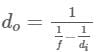to calculate the distance of the object from the lens.

Science Olympiad Test: Light- 2 - Question 2

### You are provided with a convex lens of focal length 20 cm. To obtain a real inverted and magnified image of an object, distance of object from lens must be

Detailed Solution for Science Olympiad Test: Light- 2 - Question 2

When the image formed by a convex lens, inverted and same size as the object, then the distance of the object from the lens is 2f (twice the focal length). Here,
Focal length, f = 20 cm
So, 2f = 2 × 20 cm
= 40 cm
Thus, the object should be placed at a distance of 40 cm in front of the convex lens.

Science Olympiad Test: Light- 2 - Question 3

### The linear magnification of a convex lens

Detailed Solution for Science Olympiad Test: Light- 2 - Question 3

A convex mirror would always produce an erect image, and a concave mirror may produce an inverted or an erect image depending on the position of the object placed.
Thus, independent of the sign convention, the magnification of a convex mirror is always positive, but that of a concave mirror may be both positive or negative.

Science Olympiad Test: Light- 2 - Question 4

You have caught your left ear but it appears that you have caught your right ear, it means you are standing in front of a

Detailed Solution for Science Olympiad Test: Light- 2 - Question 4

The plane mirror shows lateral inversion. The left appears right and the right appears left. So if you touch your left ear with your right hand in front of a plane mirror it will be seen in the mirror that your right ear is touched with your left hand.

Science Olympiad Test: Light- 2 - Question 5

A child is standing in front of magic mirror. He finds the image of his head bigger, the middle portion of his body of the same size and that of legs smaller. The following is the order of combinations for the magic mirror from the top.

Detailed Solution for Science Olympiad Test: Light- 2 - Question 5

As image of head is bigger, the top portion of magic mirror must be concave. The middle portion appears to be of same size, therefore, middle portion of magic mirror must be plane. As image of his legs appear smaller, the bottom of magic mirror must be convex.

Science Olympiad Test: Light- 2 - Question 6

You are given water, mustard, oil glycerine and kerosene. In which of these media a ray of light incident obliquely at same angle would bend the most?

Detailed Solution for Science Olympiad Test: Light- 2 - Question 6

Refractive index for water, mustard oil, glycerine and kerosene are:
μw​ = 1.33
μm ​= 1.47
μg​ = 1.473
μk​ = 1.44
Since refractive index of glycerine is highest, ray bends the most in case of glycerine.

Science Olympiad Test: Light- 2 - Question 7

When a ray of light is incident (coming out) from a glass slab into the air, then the reflected ray will be

Detailed Solution for Science Olympiad Test: Light- 2 - Question 7

When light rays travelling through air enters glass slab, they get refracted and bend towards the normal. Now the direction of refracted ray changes again when it comes out of the glass slab into air. Since the ray of light I know travelling from denser medium to rarer medium, it bends away from the normal.

Science Olympiad Test: Light- 2 - Question 8

A full length image of a distant tall building can definitely be seen by using

Detailed Solution for Science Olympiad Test: Light- 2 - Question 8

We know that a convex mirror forms a virtual and smaller image irrespective of position of the object. So, a full length image of a distant tall building can definitely be seen by using a convex mirror.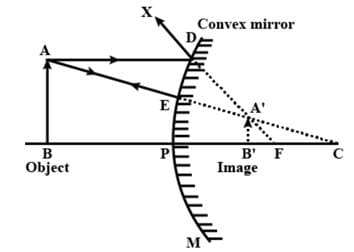Science Olympiad Test: Light- 2 - Question 9

Which of the following statement is true?

Detailed Solution for Science Olympiad Test: Light- 2 - Question 9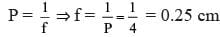Science Olympiad Test: Light- 2 - Question 10

A beam of light is incident through the holes on side A and emerges out of the holes on the side B of the box as shown in the figure. Which of the following could be inside the box?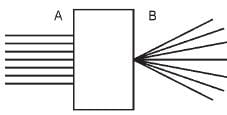Detailed Solution for Science Olympiad Test: Light- 2 - Question 10

Convex lens

• A convex lens is a lens with an outward curve.
• The thickness at the centre of a convex lens is more than the thickness at the edges of the lens.
• Convex lenses are converging lenses. They have the ability to converge a parallel beam of light into a point.
• The lens found in the human eye is a prime example of a convex lens.
• Another common example of a convex lens is the magnifying glass that is used to correct Hypermetropia or long-sightedness.
Science Olympiad Test: Light- 2 - Question 11

The power of a concave lens of focal length 2 m is

Detailed Solution for Science Olympiad Test: Light- 2 - Question 11

f = – 2 m = – 200 cm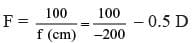Science Olympiad Test: Light- 2 - Question 12

Magnification produced by a rear view mirror fitted in which lens is

Detailed Solution for Science Olympiad Test: Light- 2 - Question 12

Convex mirrors are used in vehicles for rearview due to their wide field of view. And the convex mirror forms a virtual, erect and diminished image of the object. Therefore, magnification (m) produced by a rearview mirror fitted in vehicles is less than one.

Science Olympiad Test: Light- 2 - Question 13

In torches, search lights and headlights of vehicles, the bulb is placed

Detailed Solution for Science Olympiad Test: Light- 2 - Question 13

To create a parallel beam of light through which we can see further, the bulb in torches, search lights, and vehicle headlights is placed very close to the focus of the reflector.

Science Olympiad Test: Light- 2 - Question 14

Which of the following ray diagrams is correct for the ray of light incident on a concave mirror?

Detailed Solution for Science Olympiad Test: Light- 2 - Question 14

The ray of light incident on a concave mirror in a direction parallel to principal axis must pass through focus F on reflection from the mirror.

Science Olympiad Test: Light- 2 - Question 15

A virtual, erect and magnified image is formed by a convex lens of focal length 10 cm. The distance of object from the lens is

Detailed Solution for Science Olympiad Test: Light- 2 - Question 15

For a virtual, erect and magnified image, object lie between F and C.
If F = 10 cm, r = F/2 = 5 cm which is OC.

## Science Olympiad for Class 10

14 docs|40 tests
 Use Code STAYHOME200 and get INR 200 additional OFF Use Coupon Code
Information about Science Olympiad Test: Light- 2 Page
In this test you can find the Exam questions for Science Olympiad Test: Light- 2 solved & explained in the simplest way possible. Besides giving Questions and answers for Science Olympiad Test: Light- 2, EduRev gives you an ample number of Online tests for practice

14 docs|40 tests